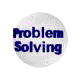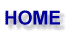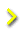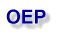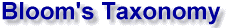Problem solving is an activity whereby a best value is determined for an unknown, which is subject to a set of constraints. To determine how to attack a problem, three problem classifications can be used to direct the investigation: by type of unknown, difficulty, and open-endedness.

The author’s preference in problem classification is that of Bloom, who classifies and identifies six problem-solving skills.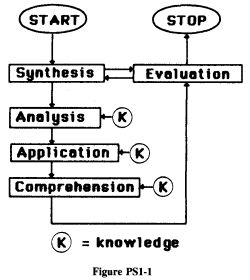1. Level of Difficulty or Skill Level

Each successive skill level calls for more advanced intellectual ability.

1. Knowledge: The remembering of previously learned material. Can the problem be solved simply by defining terms and by recalling specific facts, trends, criteria, sequences, or procedures? This level solves the type of problems such as recallin g the type of continuous flow reactor normally used for liquid-phase reactions. This is the lowest intellectual skill level. Examples of knowledge level questions are the following: Write the equations for a batch reactor and list its chara cteristics. Define. Which reactors operate at steady state? Other words used in posing knowledge questions: Who..., When..., Where..., Identify..., What formula ....

2. Comprehension: This is the first level of understanding. Given a familiar piece of information, such as a scientific principle, can the problem be solved by recalling the appropriate information and using it in conjunction with manipulation, tr anslation, interpretation, or extrapolation of the equation or scientific principle? For example, given the reactor volume [V=(v0/k)ln(CA0/CA)], can one manipulate the design equation formulas to find the effluent concentration to find the reactor volume if the inlet concentration were doubled? Compare and contrast the advantages and uses of a CSTR and a PFR. Construct a plot of NA as a function of t. Other comprehension words: ... Relate..., Show..., Distinguish..., Reconstruct..., Extrapolate... This is skill level 2.
1. Drilling the concepts
A1. Plug and chug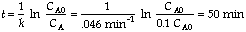2. Extrapolate
What is the time at 400 K?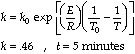3. More difficult/Think Problems
C1.Intermediate calculation
Example: 80% conversion is achieved for a first order reaction in a 1000 gal PFR, what conversion could be achieved in a CSTR for the same conditions?
C2.More than one intermediate calculation
Example: 80% conversion is achieved for a first order reaction achieved 1000 gal PFR, what conversion could be achieved in a CSTR operated at a temperature 100°C higher.

3. Application: The next higher level of understanding is recognizing which set of principles ideas, rules, equations, or methods should be applied, given all the pertinent data. Once the principle is identified, the necessary knowledge i s recalled and the problem is solved as if it were a comprehension problem (skill level 2). An application level question might be: Make use of the mole balance to solve for the concentration exiting a PFR. Other words: ... Apply..., Demonstrate..., Determine..., Illustrate....

4. Analysis: This is the process of breaking the problem into parts such that a hierarchy of subproblems or ideas is made clear and the relationships between these ideas is made explicit. In analysis, one identities missing, redundant, and contradictory information. Once the analysis of a problem is completed, the various subproblems are then reduced to problems requiring the use of skill level 3 (application). An example of an analysis question is: What conclusions did you come to after reviewing the experimental data? Other words: ... Organize..., Arrange..., What are the causes ..., What are the components....

5. Synthesis: This is the putting together of parts to form a new whole. Synthesis enters problem solving in many ways. A synthesis problem would be one requiring the type, size, and arrangement of equipment necessary to make styrene from ethylben zene. Given a fuzzy situation, synthesis is the ability to formulate (synthesize) a problem statement and/or the ability to propose a method of testing hypotheses. Once the various parts are synthesized, each part (problem) now uses the intellectual skill described in level 4 (analysis) to continue toward the complete solution. Examples of synthesis level question are: Find a way to explain the unexpected results of your experiment. Propose a research program that will elucidate the reaction mechanism. Other words: ... Speculate..., Devise..., Design..., Develop..., What alternative..., Suppose..., Create..., What would it be like..., Imagine..., What might you see....

6. Evaluation: Once the solution to the problem has been synthesized, the solution must be evaluated. Qualitative and quantitative judgments about the extent to which the materials and methods satisfy the external and internal criteria should be m ade. An example of an evaluation question is: Is the author justified in concluding that the reaction rate is the slowest step in the mechanism. Other Words: ... Was it wrong..., Will it work..., Does it solve the real problem..., Argue both sides..., Which do you like best..., Judge..., Rate....

2. Classification as Closed- or Open-Ended

As an application of the strategy outlined in Figure PS1-1, we consider a thumbnail sketch of the design of a chemical plant. Specifically, we want to produce 200 million pounds of styrene per year from ethylbenzene. First we synthesize a sequence of processing operations as shown in the synthesis level (row 1) in Figure PS1-2. In synthesis level we develop the type and arrangement of operations and equipment to produce ethylbenzene. Next we evaluate this sequence to learn if additional operations are necessary, such as a heat-exchange system following the separation sys tem or a feed purification system before the first heat exchanger. Following this cursory evaluation of our sequence, we analyze (row 2) each system (i.e. break it down into a number of subproblems). For example, Figure PS1-2 shows an analysis of t he reaction system. Here, we determine the type of reactor and catalyst to be used, the best temperature at which to carry out the reaction, the type and arrangement of reactor peripherals (e.g., heating/cooling of the reactor), and the optimum feed condi tions. After breaking down the reaction system into a number of subsystems, we proceed to the application skill level (row 3) to decide which laws or principles are to be applied to each subsystem. For example, to calculate the catalyst weight, W, we make use of the design equation (Ch. 6) for a packed-bed reactor,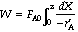In using our comprehension (level 2) skills we recall or look up the equation that gives -as a function of concentration, express concentration as a function of conversion X (Ch. 2), carry out the integration, and finally determine the catalyst weight necessary to achieve a conversion X.

3. Exercises
1. Use a specific example to explain how one could work backward from Bloom’s levels 5 through 1 to solve ill-defined open-ended problems.
2. For each of Bloom’s skill levels, construct an example that illustrates the skill used in that level (e.g., for level 1, what assumptions are used to derive the CSTR equation?).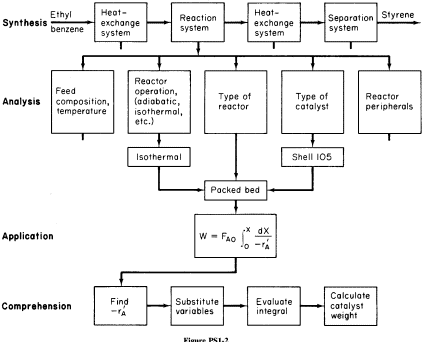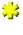Try our cobra example (check-list, web module) or move on to Closed-Ended Problems.
CRE > Thoughts > OEP > Bloom's Taxonomy